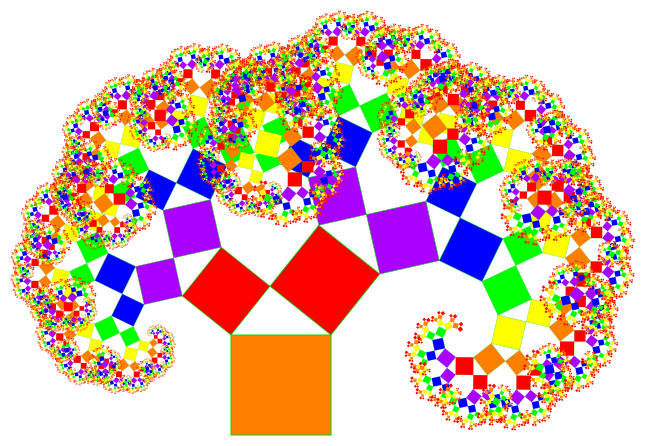# Pythagorean tripletsPythagorean triplets are triplets that satisfy the condition that $a^{2}+b^{2}=c^{2}$ such that $a. Given an unsorted array of unique integers in the text file, how many Pythagorean triplets are there?

As an explicit example, in the array $[3,7,10,6,4,8,5,11]$, there are $2$ Pythagorean Triplets, namely $(3, 4, 5)$ and $(8, 6, 10)$.

×